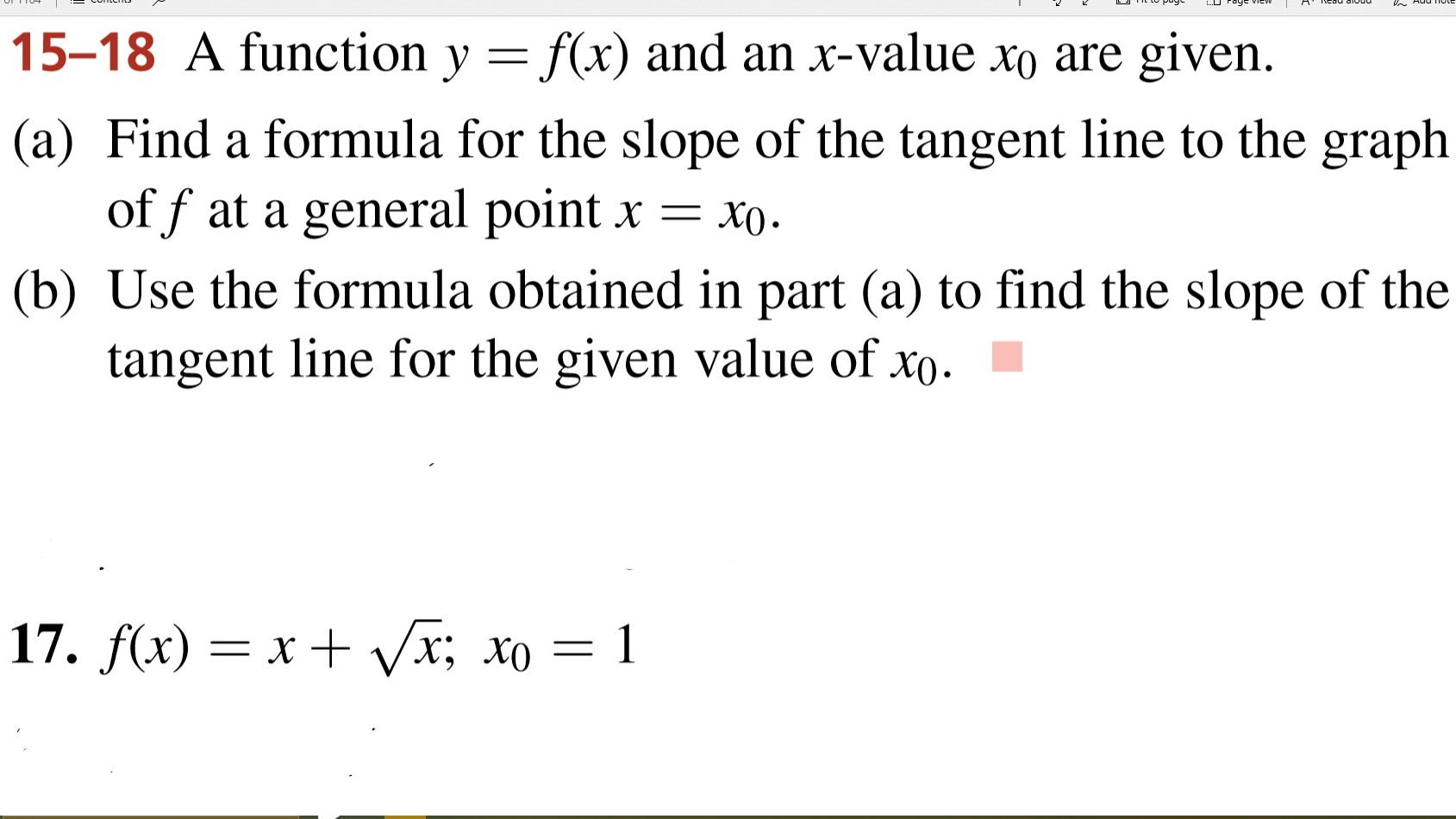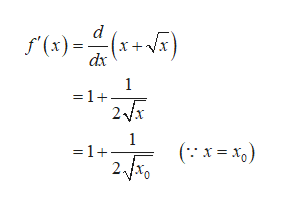15-18 A function y = f(x) and an x-value xo are given.(a) Find a formula for the slope of the tangent line to the graphof f at a general pointX(b) Use the formula obtained in part (a) to find the slope of thetangent line for the given value of xo17. f(x) xx; xo 1X

Questionhelp_outlineImage Transcriptionclose15-18 A function y = f(x) and an x-value xo are given. (a) Find a formula for the slope of the tangent line to the graph of f at a general point X (b) Use the formula obtained in part (a) to find the slope of the tangent line for the given value of xo 17. f(x) xx; xo 1 X fullscreen
Step 1

(a) The given function is

Step 2

The formula for the slope of the function f(x) at the given...help_outlineImage Transcriptionclosere)-) d f(x)= dx 1 =1 2x 1 =1- fullscreen

Want to see the full answer?

See Solution

Want to see this answer and more?

Our solutions are written by experts, many with advanced degrees, and available 24/7

See Solution
Tagged in

Derivative